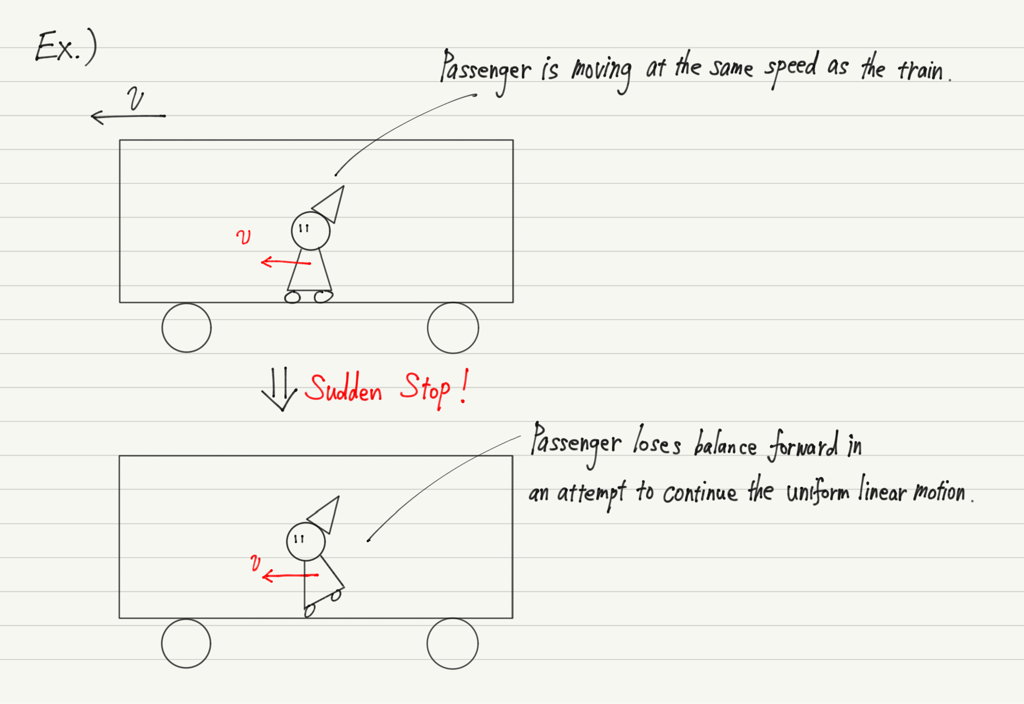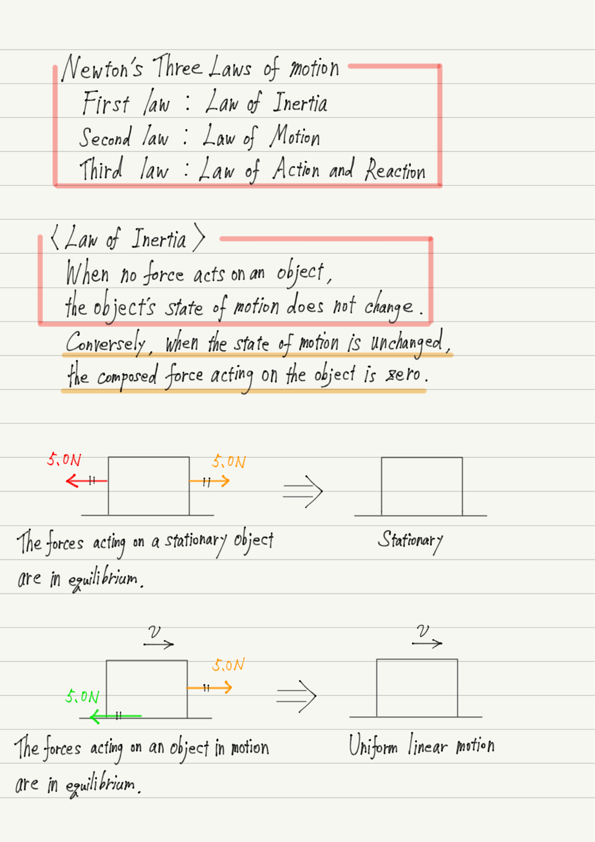# Law of Inertia

Starting with this lecture, let’s learn about Newton’s three laws of motion!

## The three laws of motion

The three laws of motion are
First law：Law of inertia
Second Law：Law of motion
Third law：Law of action and reaction
, and these are essential in studying the motion of an object!

We will look at these important laws one by one.

## Newton’s first law of motion

The Newton’s first law of motion is also known as the “Law of inertia”.

The assertion of the law of inertia can be briefly stated as, “When no force acts on an object, the object’s state of motion does not change.”

It is meaningless to memorize this law. Be able to explain the specifics of the law in your own words.
To do so, it is important to understand the conditions under which the law is valid and to be able to give specific examples of where the law applies.

## Deciphering the law of inertia

As for the first part: “no force acts an object,” this means precisely that the composed force is zero.
For example, consider an object being pulled from both sides by a force of 5 N each.
In this case, the forces cancel each other out, so it is as if no force is acted from the beginning.
The law of inertia also holds in this case.

Next, let’s look at the latter part: “the object’s state of motion does not change.”
What exactly does “state of motion” mean?

When reading textbooks, pay attention to these casual terms.
“State of motion” means whether an object is stationary or moving.

In other words, the state of motion does not change, meaning that “a stationary object remains stationary and a moving object remains moving.”

By the way, it is obvious that “a stationary object remains stationary unless a force acts on it.”
Therefore, the law of inertia requires us to pay attention to the one that says, “When no force acted, a moving object remains moving”!

This is exactly what mankind has misunderstood for a long time.
People in the past assumed that “when a force does not work, the object will eventually stop.
No, not only the people of the past! Are there any of you reading this now who also think so?

Indeed, if you slide an object on the floor, it will eventually stop.
Since we have been unconsciously observing these phenomena since we were small children, we tend to think that “moving objects will eventually stop”.

But you already know that frictional forces and air resistance are what make objects and balls stop on the floor.

The law of inertia asserts that if there is no frictional force or air resistance, a moving object will continue to move without stopping.

## Uniform linear motion, again

Let me explain “an object in motion stays in motion” a little more precisely. If the state of motion of an object does not change, it means that the speed and direction of the object in motion also do not change.

This sounds like a line I’ve heard somewhere before…?

That’s right! It’s about uniform linear motion, isn’t it!

In other words, the exact expression of the law of inertia is: “When no force acts on an object, or if it does act, it is equilibrated, a stationary object remains stationary and an object in motion has a uniform linear motion.”

Note that the converse of this law also holds true.
That is, “When an object is at stationary or in uniform linear motion, no force is acting on it.” is also correct.

## Examples of the law of inertia

Let me also give you a concrete example of the law of inertia.

A well-known example of a “stationary object remains stationary” is the tablecloth trick.
If the object below suddenly disappears, the object above will try to remain stationary and will not start moving with the object below.

The most obvious example of a “moving object remains moving” is, again, the well-known case of a train suddenly stopping, causing passengers to lose their balance in the direction of travel.Let’s find some more examples of this!

## Summary of this lecture## Next Time

We will skip the second law and look at the third law in the next lecture.

https://www.yukimura-physics.com/entry/dyn-f20

error: Content is protected !!